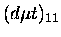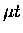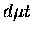Next: Resonant molecular formation Up: Muonic molecule and the Previous: Faddeev equations

## Non-Coulombic corrections

At the level of accuracy required (less than about 1 meV), the effects beyond the Coulombic, nonrelativistic point-like description of the muonic molecules are important. Because of the heavy mass of the muon, muonic hydrogen atoms and molecules are sensitive to effects such as QED vacuum polarization and the nuclear charge form factor as well as hyperfine effects. However, it is the difference between the corrections to the atomic and molecular energy levels which affects the binding energy,(37)

Because the loosely boundstates resemble aatom and a weakly bound deuteron orbiting around it, the correctionsandare similar and partly cancel each other, hence the corresponding correctionsare suppressed by a factor of about. These corrections (without hyperfine effects) amount to about a -30 meV shift (towards deeper binding) in thebinding energy.

Hyperfine structure of theandfurther introduces corrections for F=0 of +35.2 meV (S=0), and for F=1 of -8.3 meV (S=0), -14.9 meV (S=1), and -8.5 meV (S=2), where F is the total spin ofand S, that of.Next: Resonant molecular formation Up: Muonic molecule and the Previous: Faddeev equations